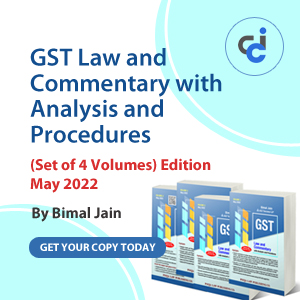Forex market  is the biggest market of the world which has a daily turnover of 4 trillion \$. It is also known as FX in short term. In forex market currencies of different countries are exchanged for the purpose of trading. There are 3 layers of forex market in India. Normally RBI works like a regulator.

a. RBI (The regulator)

b.Bank to Bank (also called interbank rate transaction)

c. Bank to customer (also called merchant rate transaction)

A customer can never directly buy or sell foreign currency. This work is done with the help of bank. Whenever the customer need to buy or sell the currency, he goes to bank and do the same.

1. So Interpretation is always done with respect to the banker’s point of view, suppose X wants to purchase \$, we won’t say he wants to buy \$, we would rather use the wording Bank is selling \$ or vice-versa.

2. There are always two market rates which are named as Bid/ Ask where Bid is the buying rate,ask is the selling rate.

3. Market always sell at higher rate and buys at low rate. And the convention of the market is to buy at lower rate and sell at higher rate.

4. Suppose 1\$ = INR 40.99/01 we will read the same as Bid is 40.99 and ask rate is 41.01

5. There  are two types of quotes are in foreign exchange first is Direct quote and second is  Indirect quote.

6. Direct quote means where price comes first and commodity comes next. Like Rs.20 per pen, here price is Rs.20 and Pen is commodity. And the same is done with foreign currency  Rs.56 per \$ means direct quote for US\$ in India. So in shortest words Direct quote can be defined as No Of units of home currency per one unit of foreign currency.

Suppose in London we have sterling pound as home currency and  \$ will be foreign currency. So  1 \$= .2 sterling pound will be called as direct quote.

Indirect quote = it will be vice-versa , here commodity will come first and price will come next. Let’s take the same example   1 \$ = 1/56 doller  this will be indirect quote. So Indirect quote is 1/direct quote.

7. Direct  quote will be called as American  and the indirect quote will be called as European  term across the globe.

8. The difference between bid and ask is called as Spread suppose Rs 44-49 is the quote given . So spread will be  Rs.4. The more stable the market will be, lesser will be the difference  between the spread.

9. Cross rate:- when we are faced with such kinds of rates which don’t involve the exact quote of what we want.  A cross rate is a rate which doesn’t involve the home currency.

Example:

One quote is SGD/INR =0.045. Another quote is euro/INR= 0.02.

Ascertain the quote for SGD in terms of Euro.

Answer :- Here we can’t calculate Euro/SGD easily. So if we want we can calculate it with:

Euro/SGD =  Euro/INR *INR/SGD = 0.02* 1/0.045  = 0.444

10. Exchange rate in forex market can be brought under 2 heads:-

a. Spot market:- where settlement is made on second working day. Suppose a transaction take place on Friday,  then Saturday and Sunday are holidays. So it means  settlement day is Monday.

b. Future market:- Forwards rate is the rate fixed  today for a future date. Suppose 1\$ = 55/56. And the bank fixes the date on ending of march as  58/59. So even if the \$ falls to 50/52.  The bank will still buy the \$ at 58 not 50.

11. We say \$ was 45/46, a year before and now its 54/55 per Rs. So we need to measure how much \$ increase and how much the value Of RS.  Fall. We do the same with appreciation and depreciation formula.

12. (Forward rate –spot rate)/Spot rate *12/months *100 .This formula is used in case of direct quote.  And we can calculate the appreciation or depreciation of  the currency.

13.(Spot rate-forward rate)/forward*12/m*100. This formula is used in case of indirect quote. And the percentage increase in doller doesn’t mean the RS.will also be decrease in the same ratio. So we calculate the same with required formulas.

14. Swap points = Forward Rate-Spot rate

 currency Spot-INR 1 month swap points Rate CAD 34.65-80 30-20 34.35-34.60 NZD 29.85-05 10-20 29.95-30.25

We will just focus on one thing, if the swap ask >swap Bid then we will add. As we had done in second example  where swap point increased from 10 to 20.

If the same don’t happen we will subtract the digits like we has done in 1st example.

Regards

Renu

*RENU SINGH *
(✩ §m!ℓ!ñġ €ม€§ fℓม!ñġ ђ♪gђ✩ )
Category Others   Report

12 Likes   84 Shares   25032 Views

### Popular ArticlesCCI Articles